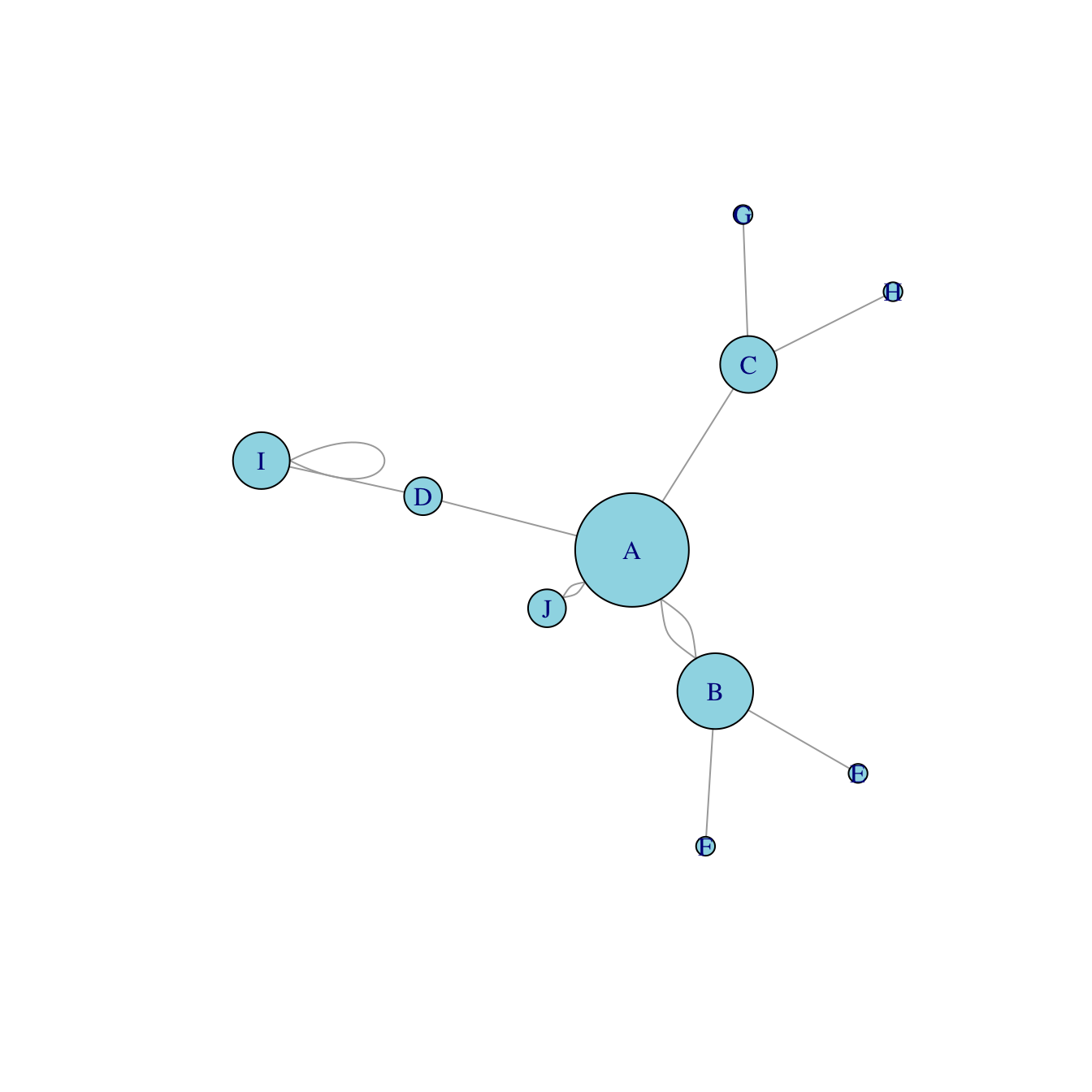# Network with node size based on edge number

This post explains how to build a network diagram where node size is proportionnal to its number of connection with other nodes. It uses R and the `igraph` package.

# Basic network diagram

It is a common task to make nodes bigger when they are heavily connected with other nodes. Indeed, it means they have an importance in the network and thus deserves to be highlighted.

The `degree()` function of the `igraph` package allows to compute the number of connection per node. It is possible to pass its result to the `vertex.size` argument of the `plot()` function to get the targeted result.``````# library
library(igraph)

# create data:
source=c("A","A", "A", "A", "A","J", "B", "B", "C", "C", "D","I"),
target=c("B","B", "C", "D", "J","A","E", "F", "G", "H", "I","I")
)

# Turn it into igraph object

# Count the number of degree for each node:
deg <- degree(network, mode="all")

# Plot
plot(network, vertex.size=deg*6, vertex.color=rgb(0.1,0.7,0.8,0.5) )``````

Related chart types

## Contact

This document is a work by Yan Holtz. Any feedback is highly encouraged. You can fill an issue on Github, drop me a message on Twitter, or send an email pasting yan.holtz.data with gmail.com.